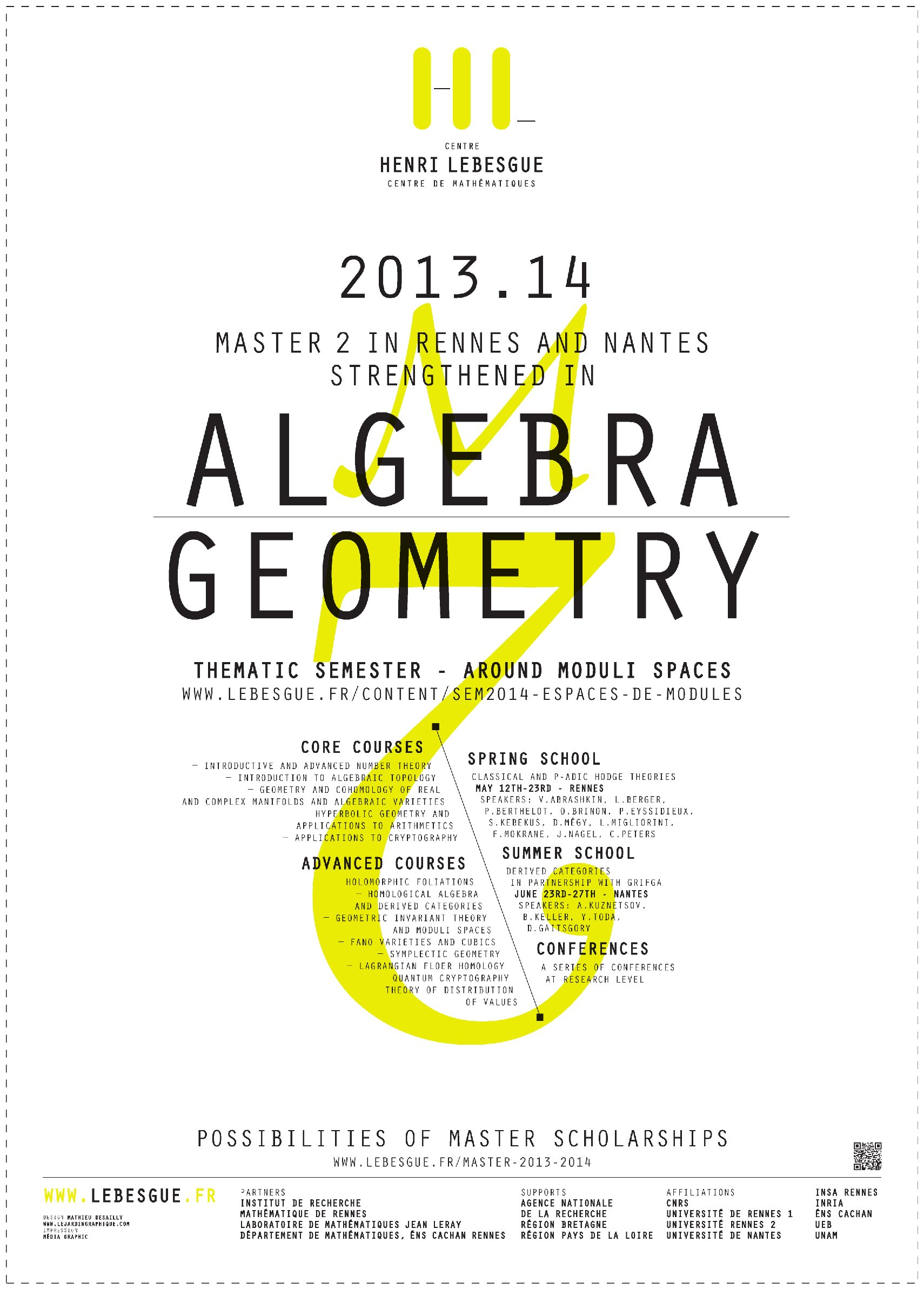The universities of Nantes and Rennes propose a special Master program strengthened in algebra and geometry related to the topic of the thematic semester.

The Labex offers Master scholarships as well. Applications here.

## Courses

### Core courses

• Introductive and advanced number theory
• Introduction to algebraic topology
• Geometry and cohomology of real and complex manifolds and algebraic varieties (including scheme theory)
• Hyperbolic geometry and applications to arithmetics
• Applications to cryptography

• Holomorphic foliations
• Homological algebra and derived categories
• Geometric invariant theory and moduli spaces
• Fano varieties and cubics
• Symplectic geometry
• Lagrangian Floer homology
• Quantum cryptography
• Theory of distribution of values

### Doctoral courses

• Homological algebra and derived categories
• Geometric invariant theory and moduli spaces